# Hacking a Bluetooth Toy Car

We're learning how to "hack" a bluetooth toy car by building a custom remote for it. using arduino and a gyroscope.

IntermediateFull instructions provided3,032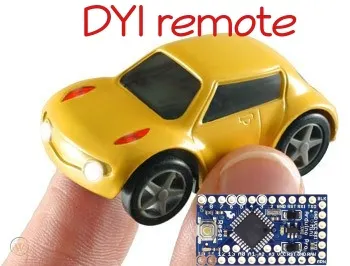## Things used in this project

### Hardware components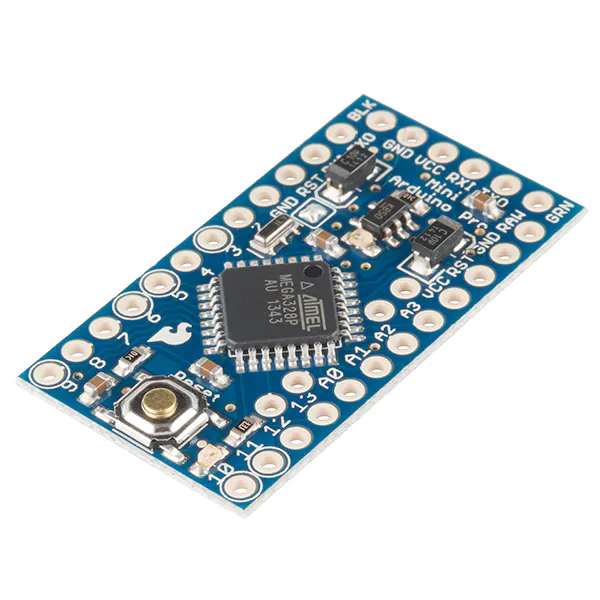SparkFun Arduino Pro Mini 328 - 5V/16MHz
×1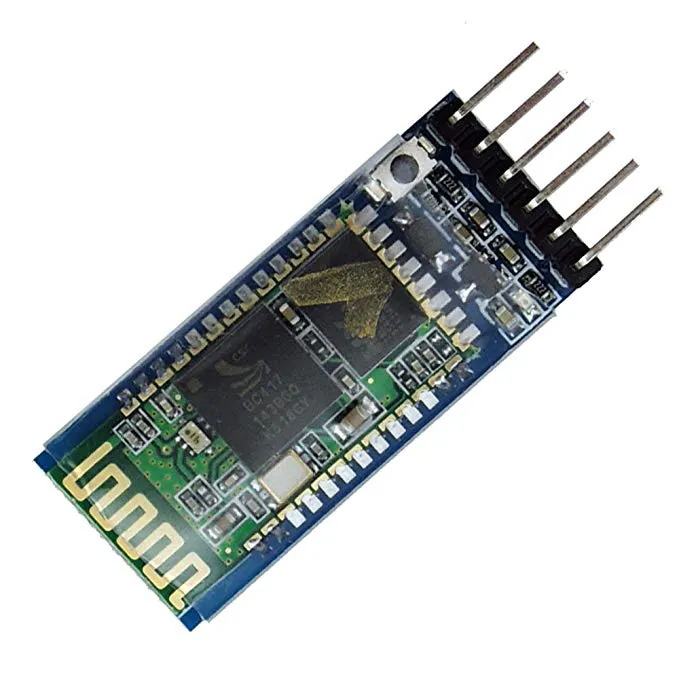HC-05 Bluetooth Module
×1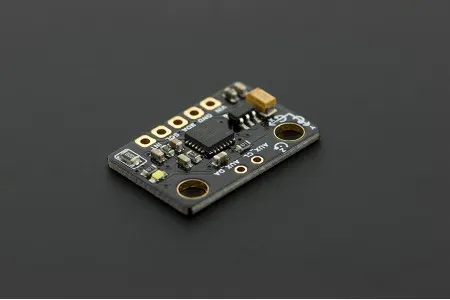DFRobot 6 DOF Sensor - MPU6050
×1×1Jumper wires (generic)
×1

### Hand tools and fabrication machines

 Arduino IDE
 USB to serial FTDI adapter FT232RL
 Android phone
 Android studio

## Schematics

### Fritznig skematic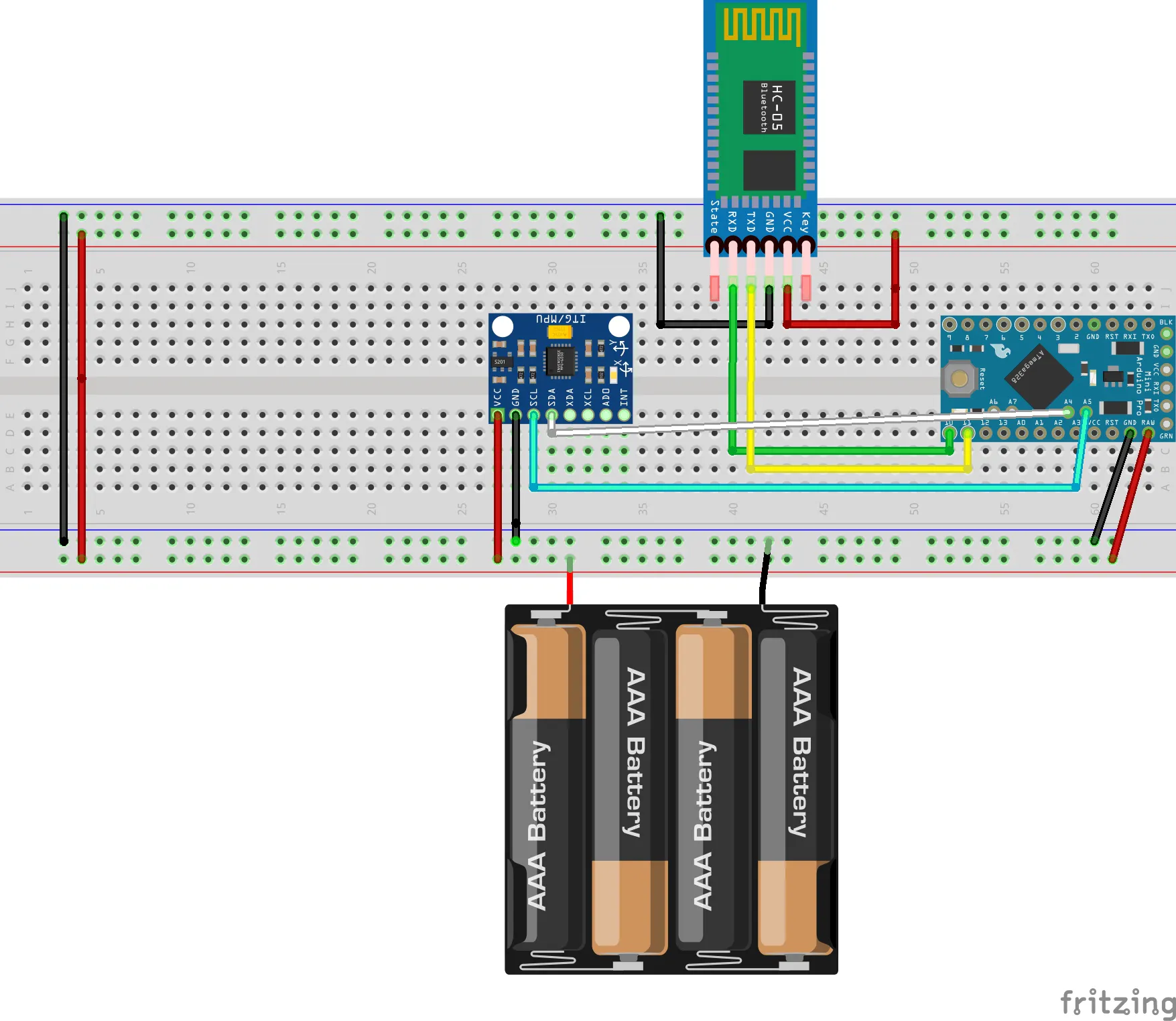### Arduino repository

For this project navigate to: /projects/zen_wheels_remote

## Code

### Code snippet #4

Plain text
```private final int value_convert_out(int value) {
boolean negative = false;
if (value < 0) {
negative = f6D;
}
int value2 = value & 63;
if (negative) {
return value2 | 64;
}
return value2;
}
```

### Code snippet #6

Plain text
```/** * Libraries:
*  https://github.com/jrowberg/i2cdevlib

*  https://github.com/jrowberg/i2cdevlib

*/
#include "I2Cdev.h"
#include "MPU6050_6Axis_MotionApps20.h"
#include "Wire.h"
#include "SoftwareSerial.h"const int MAX_ANGLE = 45;
const byte commandStering = 129;
const byte commandSpeed = 130;bool initialization = false;  // set true if DMP init was successful
uint8_t mpuIntStatus;   // holds actual interrupt status byte from MPU
uint8_t devStatus;      // return status after each device operation (0 = success, !0 = error)
uint16_t packetSize;    // expected DMP packet size (default is 42 bytes)
uint16_t fifoCount;     // count of all bytes currently in FIFO
uint8_t fifoBuffer; // FIFO storage buffer
Quaternion q;           // [w, x, y, z]         quaternion container
VectorFloat gravity;    // [x, y, z]            gravity vector
float ypr;           // [yaw, pitch, roll]   yaw/pitch/roll container and gravity vector
volatile bool mpuInterrupt = false;     // indicates whether MPU interrupt pin has gone highunsigned long lastPrintTime, lastMoveTime = 0;SoftwareSerial BTserial(10, 11);
MPU6050 mpu;void setup()
{
Serial.begin(9600);
BTserial.begin(38400);
Serial.println("Program started");
initialization = initializeGyroscope();
}void loop() {
if (!initialization) {
return;
}
mpuInterrupt = false;
mpuIntStatus = mpu.getIntStatus();
fifoCount = mpu.getFIFOCount();
if (hasFifoOverflown(mpuIntStatus, fifoCount)) {
mpu.resetFIFO();
return;
}
if (mpuIntStatus & 0x02) {
while (fifoCount < packetSize) {
fifoCount = mpu.getFIFOCount();
}
mpu.getFIFOBytes(fifoBuffer, packetSize);
fifoCount -= packetSize;
mpu.dmpGetQuaternion(&q, fifoBuffer);
mpu.dmpGetGravity(&gravity, &q);
mpu.dmpGetYawPitchRoll(ypr, &q, &gravity);
steer(ypr * 180/M_PI, ypr * 180/M_PI, ypr * 180/M_PI);
}
}/*
* Receives angle from 0 to 180 where 0 is max left and 180 is max right
* Receives speed from -90 to 90 where -90 is max backwards and 90 is max forward
*/
void moveZwheelsCar(byte angle, int speed)
{
if (millis() - lastMoveTime < 100) {
return;
}
byte resultAngle;
int resultSpeed;
if (angle >= 90) {
resultAngle = map(angle, 91, 180, 1, 60);
} else if (angle < 90) {
resultAngle = map(angle, 1, 90, 60, 120);
}
if (speed > 0) {
resultSpeed = map(speed, 0, 90, 0, 60);
} else if (speed < 0) {
resultSpeed = map(speed, 0, -90, 120, 60);
}
Serial.print("actualAngle=");Serial.print(angle);Serial.print("; ");
Serial.print("actualSpeed=");Serial.print(resultSpeed);Serial.println("; ");
BTserial.write(commandStering);
BTserial.write(resultAngle);
BTserial.write(commandSpeed);
BTserial.write((byte) resultSpeed);
lastMoveTime = millis();
}void steer(int x, int y, int z)
{
x = constrain(x, -1 * MAX_ANGLE, MAX_ANGLE);
y = constrain(y, -1 * MAX_ANGLE, MAX_ANGLE);
z = constrain(z, -MAX_ANGLE, MAX_ANGLE);
int angle = map(y, -MAX_ANGLE, MAX_ANGLE, 0, 180);
int speed = map(z, -MAX_ANGLE, MAX_ANGLE, 90, -90);
printDebug(x, y, z, angle, speed);
moveZwheelsCar(angle, speed);
}void printDebug(int x, int y, int z, int angle, int speed)
{
if (millis() - lastPrintTime < 1000) {
return;
}
Serial.print("z=");Serial.print(x);Serial.print("; ");
Serial.print("y=");Serial.print(y);Serial.print("; ");
Serial.print("z=");Serial.print(z);Serial.print("; ");
Serial.print("angle=");Serial.print(angle);Serial.print("; ");
Serial.print("speed=");Serial.print(speed);Serial.println("; ");
lastPrintTime = millis();
}bool initializeGyroscope()
{
Wire.begin();
mpu.initialize();
Serial.println(mpu.testConnection() ? F("MPU6050 connection successful") : F("MPU6050 connection failed"));
devStatus = mpu.dmpInitialize();
mpu.setXGyroOffset(220);
mpu.setYGyroOffset(76);
mpu.setZGyroOffset(-85);
mpu.setZAccelOffset(1788);
if (devStatus != 0) {
Serial.print(F("DMP Initialization failed (code "));Serial.println(devStatus);
return false;
}
mpu.setDMPEnabled(true);
Serial.println(F("Enabling interrupt detection (Arduino external interrupt 0)..."));
mpuIntStatus = mpu.getIntStatus();
Serial.println(F("DMP ready! Waiting for first interrupt..."));
packetSize = mpu.dmpGetFIFOPacketSize();

return true;
{
mpuInterrupt = true;
}boolean hasFifoOverflown(int mpuIntStatus, int fifoCount)
{
return mpuIntStatus & 0x10 || fifoCount == 1024;
}
```

### Github

https://github.com/danionescu0/arduino

### Github

https://github.com/jrowberg/i2cdevlib

### Github

https://github.com/pybluez/pybluez

## Credits

### danionescu

10 projects • 50 followers
I'm an electronics enthusiast, passionate about science, and programming.I like the challenges involved with building things from scratch.